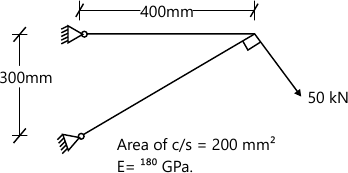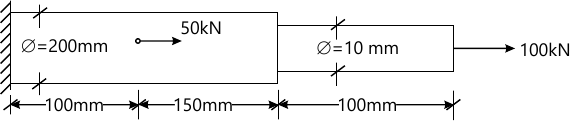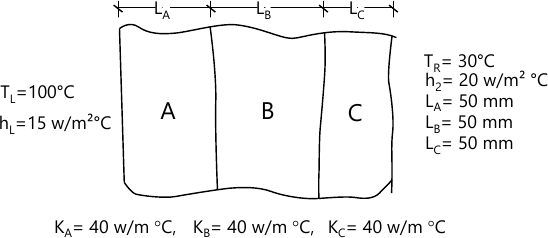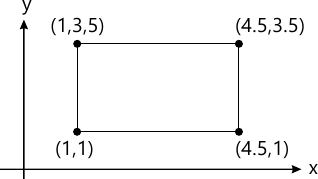MORE IN Finite Element Analysis
MU Mechanical Engineering (Semester 8)
Finite Element Analysis
December 2014
Total marks: --
Total time: --
INSTRUCTIONS
(1) Assume appropriate data and state your reasons
(2) Marks are given to the right of every question
(3) Draw neat diagrams wherever necessary

1 (a) (i) Explain different types of nodes with suitable examples.
5 M
1 (a) (ii) Give any two fields of engineering problems governed by the second-order differential equation and its parameters.
5 M
1 (b) Use Gaussin Legandre Quadrature Method and for the integral in two dimension (2×2) and verify exactness by an analytical integration. $I=\int^{+1}_{-1}\int^{+1}_{-1} (2x^4 +3y^2 +x^3y)dx \ dy$
10 M

2 (a) Solve the following differential equation by using Galerkin's method. $\dfrac {d^2u}{dx^2}=f_c$ where the domain is changing from (0≤x≤L) and B.C.'s of the problem: $\dfrac {du}{dx}(0)=0; \ \dfrac {du}{dx}(L)=0$
10 M
2 (b) Solve the following differential equation using (i) subdomain (ii) collocation $\dfrac{d}{dx} \left ( x \dfrac {dy}{dx} \right ) -4x=10 \ \ \ \1\le x \le 2$ subject to y(1)=y(2)=0
10 M

3 (a) (i) Explain 'h' and 'p' method in FEM.
4 M
3 (a) (ii) Explain at which are the conditions for the Rayleigh Ritz and Galerkin methods yield the same solution.
4 M
3 (b) Develop the Finite Element equation for the most general element using Rayleigh-Ritz method for the mathematical model given $\dfrac {d}{dx} \left ( AE \dfrac {du}{dx} \right ) =0 \ \ \ \ 0\le x \le 12 \ cms$ Take linear elements. Use Lagrange's linear shape functions. Use the following data to solve Global equation. Use three elements. A=0.1 m2, for each element, E=100 GPa for each element, u(0)=0 and u(12), p=external force=10 kN. Find displacements at nodes and forces. Explain each step clearly.
12 M

4 (a) Determine the shape functions for Euler Bernoulli Beam.
8 M
4 (b) Analyse the following Truss completely for reactions, stress and strains.12 M

5 (a) Find the displacement, stresses and strain in the elements of stepped bar as shown in figure. Take E=200 Gpa.10 M
5 (b) Find the temperature at interfaces and heat transfer per unit area through the wall.10 M

6 (a) (i) Write down the polynomial expression for quadratic rectangular element for the following:-
Lagrange Element
Serendipity Element
4 M
6 (a) (ii) Using serendipity concept, find the shape function for the eight nodes rectangular element.
4 M
6 (b) Calculate the linear interpolation function for the linear rectangular elements shown in figure.8 M

7 (a) (i) Write a notes on PATCH Test and Jacobian Matrix.
5 M
7 (a) (ii) Explain compatibility and convergence criteria.
5 M
7 (b) Find the natural frequencies of the axial vibrations of a fixed free bar of uniform cross section of 50 mm2 and length of 1 meter using consistent and lumped mass matrix and compare the natural frequencies with exact frequencies. Take E=200 GPa and density=7860 kg/m3. Take two linear elements.
10 M

More question papers from Finite Element Analysis